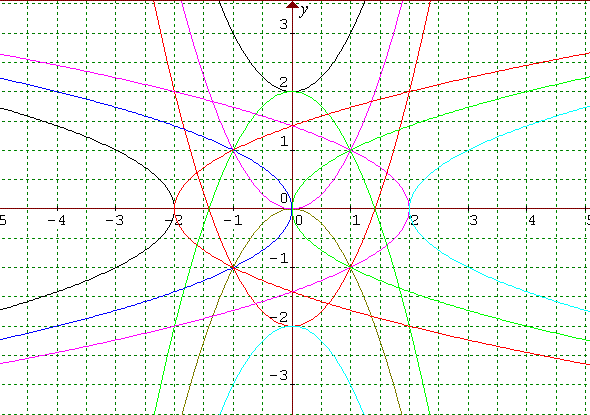#### You may also like### Cubic Spin

Prove that the graph of f(x) = x^3 - 6x^2 +9x +1 has rotational symmetry. Do graphs of all cubics have rotational symmetry?### Sine Problem

In this 'mesh' of sine graphs, one of the graphs is the graph of the sine function. Find the equations of the other graphs to reproduce the pattern.### Parabolic Patterns

The illustration shows the graphs of fifteen functions. Two of them have equations y=x^2 and y=-(x-4)^2. Find the equations of all the other graphs.

# More Parabolic Patterns

##### Age 14 to 18Challenge LevelThe illustration shows the graphs of twelve functions. Three of them have equations

$y = x^2$
$x = y^2$
$x = -y^2 + 2$

Use a graphic calculator or a graph drawing program to sketch these three graphs and then to locate them in this illustration. Use the clues given in this information to help you to find the equations of all the other graphs and to draw the pattern of 12 graphs for yourself. For your solution send in the equations you have found with an explanation of how you did it.